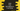# Java program to find the third largest number in an unsorted array### Java program to find the third largest number in an unsorted array :

In this Java programming tutorial, we will learn how to find the third largest number in an array. The program will pass one unsorted array to a function,the function will find the third largest number in the array and then it will return it back to the main function. We will learn two different methods to solve this problem.

Let’s have a look at the program:

### Method 1 : using one single loop :

``````public class New {
//2
private static int findThirdLargest(int[] array) {
//3
int first, second, third;
//4
first = second = third = array;
//5
for (int i = 1; i < array.length; i++) {
//6
if (array[i] > first) {
third = second;
second = first;
first = array[i];
} else if (array[i] > second) {
//7
third = second;
second = array[i];
} else if (array[i] > third) {
//8
third = array[i];
}
}
//9
return third;
}

public static void main(String[] args) {
//1
int a[] = {4, 3, 2, 11, 23, 3, 44, 8, 93, 2, 34, 7, 8, 9};
System.out.println("Third largest number is " + findThirdLargest(a));
}
}``````

### Explanation :

The commented numbers in the above program denote the step number below :

1. The integer array a contains few random numbers. We will pass this array to a different function to find out the third largest number. This function will return the third largest number and print out the result.
2. findThirdLargest function takes an array of integer as argument and return the third largest number.
3. Create three integer variables first,second and third to hold the first, second and third largest number in the array.
4. First, assign the value of first number of the array to first, second and third.
5. Run one for loop to scan each number of the array.
6. Check if the number is more than first or not.If yes, set the value of second to third, value of first to second and then set the new largest value to first. Basically, we are updating all values of first,second and third.
7. Again, if the current number is more than second, update both second and third value.
8. Finally, if the current number is more than only third, update only third value.
9. Finally return the third value.
10. 10.

### Method 2 : Using sorting :

``````import java.util.Arrays;

public class New {
private static int findThirdLargest(int[] array) {
Arrays.sort(array);
return array[array.length - 3];
}

public static void main(String[] args) {
int b[] = {4, 3, 2, 11, 23, 3, 44, 8, 93, 2, 34, 7, 8, 9};
System.out.println("Third largest number is " + findThirdLargest(b));
}
}``````

In this program, we are first sorting the array using Arrays.sort() method. Then the function is returning the third largest number i.e. of position array.length - 3. Both of these above program will print 34